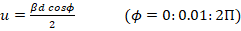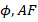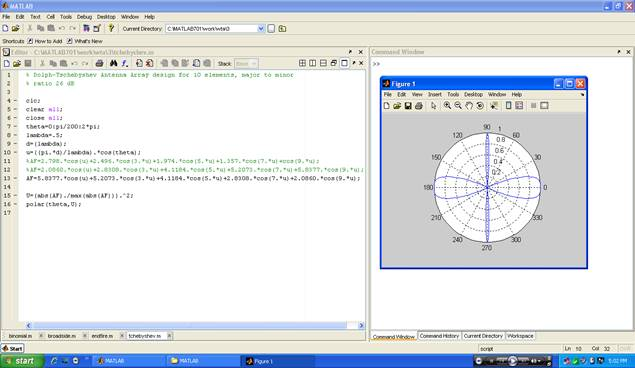EXPERIMENT NO 10.

Dolph-Tchebyshev Array Antenna

Practical Session Plan

 Time ( min) Content Learning Aid / Methodology Faculty Approach Typical Student Activity Skill / Competency Developed 10 Relevance and significance of experiment Chalk & Talk , Presentation Introduces, Facilitates, Monitors Listens, Participates, Discusses Knowledge, Communication, intrapersonal 20 Explanation of experiment Chalk & Talk , Presentation Introduces, Facilitates, Explains Listens Knowledge, Communication, intrapersonal, Application 10 Calculations N/A Explains, Monitors Participates, Discusses Knowledge, Communication, Intrapersonal, Application 50 Programming Demonstration Explains, Monitors Participates, Discusses Debate, comprehension, Hands on experiment 10 Debugging & Simlating Demonstration Explains, Monitors Participates, Discusses Hands on experiment 10 Results and conclusions Keywords Lists, Facilitates Listens, Participates, Discusses Knowledge, Communication, intrapersonal, Comprehension 10 Oral Question Discussion Discussion Discussion Identifies & Participates Knowledge Intrapersonal

Title: Dolph-Tchebyshev Array Antenna

Objectives: Model the Dolph Tchebyshev array to analyze the performance parameters

Aim:

Â· To sketch the radiation pattern of Dolph Tchebyshev array with uniform spacing & amplitude

Â· To compute performance parameters

Tool used: MATLAB.

Theory:

Plot (AF)dB Vs, polar (,(A)dB) Another array, with many practical applications, is the Dolph-Tschebyscheff array. The method was originally introduced by Dolph and investigated afterward by others. It is primarily a compromise between uniform and binomial arrays. Its excitation coefficients are related to Tschebyscheff polynomials. A Dolph-Tschebyscheff array with no side lobes (or side lobes of âˆ’âˆž dB) reduces to the binomial design.

The recursion formula for Tschebyscheff polynomials isIt can be used to find one Tschebyscheff polynomial if the polynomials of the previous two orders are known. Each polynomial can also be computed usingThe following properties of the polynomials are of interest:

1. All polynomials, of any order, pass through the point (1, 1).

2. Withinthe range âˆ’1 â‰¤ z â‰¤ 1, the polynomials have values within âˆ’1 to +1.

3. All roots occur within âˆ’1 â‰¤ z â‰¤ 1, and all maxima and minima have values of +1 an dâˆ’1, respectively.

Since the array factor of an even or odd number of elements is a summation of cosine terms whose form is the same as the Tschebyscheff polynomials, the unknown coefficients of the array factor can be determined by equating the series representing the cosine terms of the array factor to the appropriate Tschebyscheff polynomial. The order of the polynomial should be one less than the total number of elements of the array.

Algorithm for Dolph Tchebyscheff Antenna:

1. Read the values of n and f

2. Calculate Î», Î².

3. Read the value of â€˜dâ€™ such that d< Î»/2.

4. Calculate5. Calculate Tchebyscheff polynomials using Chebwin(column vector of N size).

6. Take transpose of column vector.

7. Check the condition whether N is even or odd using MOD operation.

8. Calculate coefficient A(x) of AF using Tchebyscheff polynomials (1 to N/2).

9. Calculate AF.

10. Plot AF Vs , polar().

11. Calculate AF in dB.

12.

MATLAB program:

% Dolph-Tschebyshev Antenna Array design for 10 elements, major to minor

% ratio 26 dB

clc;

clear all;

close all;

theta=0:pi/200:2*pi;

lambda=.5;

d=(lambda);

u=((pi.*d)/lambda).*cos(theta);

%AF=2.798.*cos(u)+2.496.*cos(3.*u)+1.974.*cos(5.*u)+1.357.*cos(7.*u)+cos(9.*u);

%AF=2.0860.*cos(u)+2.8308.*cos(3.*u)+4.1184.*cos(5.*u)+5.2073.*cos(7.*u)+5.8377.*cos(9.*u);

AF=5.8377.*cos(u)+5.2073.*cos(3.*u)+4.1184.*cos(5.*u)+2.8308.*cos(7.*u)+2.0860.*cos(9.*u);

U=(abs(AF)./max(abs(AF))).^2;

polar(theta,U);

Results:Conclusion:

Dolph-Tchebysheff Array is based on Tchebysheff polynomials. It has narrowest main lobe for the given side lobe sides. The current distribution reduces the pattern which is similar to Tchebysheff polynomials.

Upon completion of experiment students will be able to :

Students will able to model the Dolph Tchebyshev, compute the performance parameters and compare the performance parameters with standard parameters